# Class 12 Chemistry: CBSE Sample Question Paper- Term I (2021-22)- 4 Notes | Study Sample Papers for Class 12 Medical and Non-Medical - Class 12

## Class 12: Class 12 Chemistry: CBSE Sample Question Paper- Term I (2021-22)- 4 Notes | Study Sample Papers for Class 12 Medical and Non-Medical - Class 12

The document Class 12 Chemistry: CBSE Sample Question Paper- Term I (2021-22)- 4 Notes | Study Sample Papers for Class 12 Medical and Non-Medical - Class 12 is a part of the Class 12 Course Sample Papers for Class 12 Medical and Non-Medical.
All you need of Class 12 at this link: Class 12

Class-XII
Time: 90 Minutes
Max. Marks: 35

General Instructions :

1. The Question Paper contains three sections.
2. Section A has 25 questions. Attempt any 20 questions.
3. Section B has 24 questions. Attempt any 20 questions.
4. Section C has 6 questions. Attempt any 5 questions.
5. All questions carry equal marks.
6. There is no negative marking.

Section - A

Q.1: Anoxia is a condition related to people who ?
(a) are divers
(b) climb high altitudes
(c) live in snowy region
(d) are obese

Anoxia is a condition which leads to low concentrations of oxygen in the blood and tissues of people living at high altitudes or climbers. Low blood oxygen causes climbers to become weak and unable to think clearly, symptoms of a condition known as anoxia.

Q.2: _____________ is an antifreeze used in cars for cooling the engine.
(a) ethane glycol
(b) methylene glycol
(c) ethylene glycol
(d) none of the above

Ethylene glycol is an antifreeze used in cars for cooling the engine.

Q.3: The correct order for increase in density is:
(a) n-C3H7I < n-C3H7Cl < n- C3H7Br
(b) n-C3H7I < n-C3H7Br < n-C3H7Cl
(c) n-C3H7Cl < n- C3H7Br < n-C3H7
(d) n- C3H7Br < n-C3H7I < n-C3H7Cl

It depends on conc. of only one reactant, t-butyl chloride.

Q.4: Used as a diluent for oxygen in modern diving apparatus:
(a) neon
(b) liquid helium
(c) argon
(d) liquid nitrogen

Liquid helium is used as a diluent for oxygen in modern diving apparatus because of its very low solubility in blood.

Q.5: Choose the correct product for the given reaction: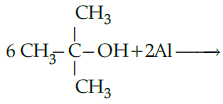(a)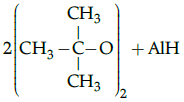(b)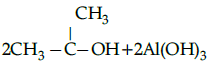(c)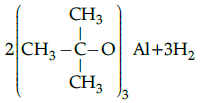(d)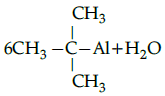Alcohols react with active metals such as aluminium to yield corresponding alkoxides and hydrogen.

Q.6: Which of the following do not show allotropy ?
(a) Nitrogen
(b) Phosphorus
(c) Arsenic
(d) All of the above

Except nitrogen, all the other group 15 elements show allotropy.

Q.7: In which pair most efficient packing is present?
(a) hcp and bcc
(b) hcp and ccp
(c) bcc and ccp
(d) bcc and simple cubic

hcp and ccp has 74% packing efficiency i.e. 74% of the space in filled.

Q.8: When there are no unpaired electrons the substance shows __________ while with one or more unpaired electrons, the substance shows ______________:
(a) diamagnetism, paramagnetism
(b) paramagnetism, diamagnetism
(c) ferromagnetism, diamagnetism
(d) diamagnetism, ferromagnetism

The substances having paired electrons are diamagnetic in nature while those having unpaired electrons are paramagnetic in nature.

Q.9: The dinitrogen gas after preparation is passed through aqueous sulphuric acid containing potassium dichromate to remove:
(a) NO2 and H2SO4
(b) NO and HCl
(c) NO and HNO3
(d) NO2 and HNO3

NO and HNO3 are the impurities formed during the preparation of dinitrogen and are removed by passing the gas through aqueous sulphuric acid containing potassium dichromate.

Q.10: Which of the following shows nucleophilic substitution by SN1 mechanism:
(a)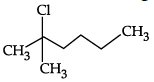(b)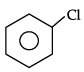(c)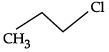(d)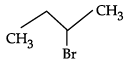As it is tertiary halide, the carbo cation formed will be more stable.

Q.11: Aniline on treatment with nitrous acid at 273-278 K gives:
(a) benzene amide
(b) benzene diazonium chloride
(c) benzene peroxide
(d) benzene diazonium oxide

Benzene diazonium chloride is formed which is hydrolysed to phenols by warming with water.

Q.12: Supersonic jet aeroplanes are responsible for depleting the conc. of ozone layer because:
(a) they emit nitrogen oxides.
(b) nitrogen oxides readily combine with ozone.
(c) none of the above
(d) both (a) and (b)

Nitrogen oxides emitted from the exhaust systems of supersonic jet aeroplanes is slowly depleting the concentration of the ozone layer in the upper atmosphere as nitrogen oxides (particularly nitrogen monoxide) combine very rapidly with ozone.

Q.13: When hydrochloric acid reacts with ammonia __________ is produced:
(a) white fumes of NH4OH
(b) white fumes of NH4Cl
(c) hydrogen gas
(d) chlorine gas

Hydrochloric acid reacts with ammonia and ionise into ammonium ion and chloride ion which further combines to form white fumes of NH4Cl.

NH3(aq) + HCl(aq) → NH4 +(aq)+ Cl- (aq) NH4 +(aq) + Cl- (aq) → NH4Cl(g)

Q.14: When measured along different directions in the same crystals some properties like refractive index and electrical resistance have different values. This nature of solids are known as:
(a) Anisotropic
(b) Isotropic
(c) Pseudo solids
(d) None of the above

Q.15: Which of the following is incorrectly paired ?
(a) chloramphenicol – dengue fever
(b) chloroquine – malaria
(c) halothane – surgery
(d) thyroxine – goiter

Chloramphenicol is helpful in treatment of typhoid fever.

Q.16: The acid strength of alcohol is:

(a)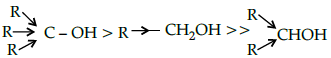(b)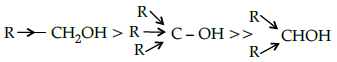(c)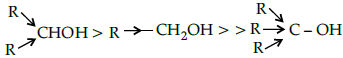(d)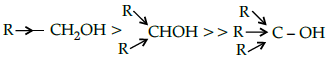Acid strength decreases in the order given.

Q.17: Chlorobenzene is fused with NaOH at 623 K and 320 atmospheric pressure. Sodium phenoxide thus obtained undergoes _______ to obtain phenol.
(a) hydrolysis
(b) hydrogenation
(c) acidification
(d) neutralisation

Acidification using HCl converts sodium phenoxide to phenol.

Q.18: In SN2 reactions tertiary halides are least reactive due to:
(a) halide group
(b) three hydrogen atoms
(c) three methyl group
(d) conc. of halide group

Methyl groups are bulky group which hinders the approaching nucleophile.

Q.19: Which of the following is the correct order ?
(a) N2O –basic oxide
(b) Al2O3 – neutral oxide
(c) Na2O - amphoteric oxide
(d) CrO3 – acidic oxide

CrO3 is an acidic oxide. This is because metals in high oxidation state form acidic oxide.

Q.20: The correct order of increasing the reactivity of C—X bond towards nucleophile in following compounds: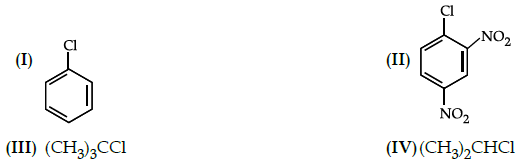(a) IV < III < II < I
(b) I < II < III < IV
(c) III < II < I < IV
(d) II < III < I < IV

III is most reactive due to stability of tertiary carbo cation.
-NO2 (electron withdrawing groups increases nucleophilic substitution reaction.

Q.21: Which of the following is an ambident nucleophile ?
(i) cyanides
(ii) hydroxyl
(iii) nitrites
(a) (i), (ii)
(b) (i), (ii), (iii)
(c) (i), (iii)
(d) (ii), (iii)

As it can link through carbon or nitrogen atom (as in case of cyanide) or through nitrogen or oxygen atom as in nitrites, it is called as ambident nucleophile.

Q.22: The presence of strong hydrogen bonding in H2O as compare to H2S is due to:
(a) small size of oxygen
(b) high electronegativity in O
(c) low electronegativity in O
(d) both (a) and (b)

The anomalous behaviour like, strong hydrogen bonding in H2O is due to small size and high electro-negativity of oxygen.

Q.23: Which of the following solutions possess lowest freezing point?
(a) 1 M KCl
(b) 1 M K2SO4
(c) 1 M glucose
(d) 1 M Urea

Depression in freezing point is colligative property and it depends upon the number of particles or ions. Greater the number of ions in solution, lesser will be the freezing point.
Since, 1 M K2SO4 dissociates into three ions, so, its freezing point will be lowest.

Q.24: Which of the following compounds does not exist ?

(a) NeF2
(b) XeF2
(c) XeO3
(d) XeOF4

NeF2 does not exist because the first and second ionization enthalpies of Ne as very light.

Q.25: Ethanol on heating with conc. sulphuric acid at 170˚C gives:
(a) Ethyl hydrogen sulphate
(b) Diethyl ether
(c) Diethyl sulphate
(d) Ethylene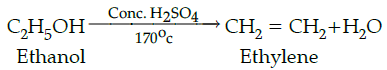Section - B

Q.26: How much ethyl alcohol must be added to 1 litre of water so that the solution will freeze at– 14°C ?

(Kf for water = 1.86°C/mol).
(a) 7.5 mol
(b) 8.5 mol
(c) 9.5 mol
(d) 10.5 mol

7.5 mol

ΔTf = Kf m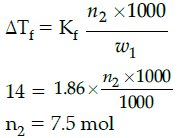Q.27: Which reagents are required for one step conversion of chlorobenzene to toluene?
(a) CH3Cl / AlCl3
(b) CH3Cl, Na, Dry ether
(c) CH3Cl/Fe dark
(d) NaNO2/ HCl /0-5°C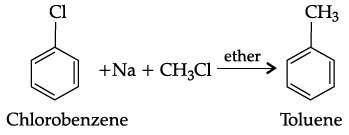Q.28: On partial hydrolysis, XeF6 gives:
(a) XeO3 +4HF
(b) XeO2F + HF
(c) XeOF4+ 2HF
(d) XeO2F2 + 4HF

The reaction involved in the partial hydrolysis is: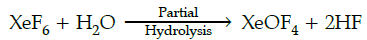Q.29: Which one of the following statement is correct about sucrose:
(a) It can reduce tollen’s reagent however cannot reduce fehling’s reagent
(b) It undergoes mutarotation like glucose and fructose
(c) It undergoes inversion in the configuration on hydrolysis
(d) It is laevorotatory in nature.

It undergoes inversion in the configuration on hydrolysis.

Q.30: Phenol does not undergo nucleophilic substitution reaction easily due to:
(a) Acidic nature of phenol
(b) Partial double bond character of C-OH bond
(c) Partial double bond character of C-C bond
(d) Instability of phenoxide ion

Phenol does not undergo nucleophilic substitution reaction due to partial double bond character of C-OH bond. It is due to the fact that the oxygen gets attached to the carbon on the benzene ring acquires a partial double bond character by means of resonance(+R) effect, making it difficult to break.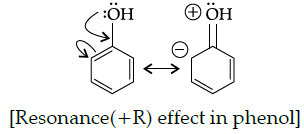Q.31: Which of the following has highest ionisation enthalpy?
(a) Nitrogen
(b) Phosphorus
(c) Oxygen
(d) Sulphur

High IE of N is because of smallest size in the group and completely half - filled p-subshell.

Q.32: Metal M ions form a ccp structure. Oxide ions occupy ½ octahedral and ½ tetrahedral voids. What is the formula of the oxide?
(a) MO
(b) MO2
(c) MO3
(d) M2O3

Metal M ions form ccp structure. Let number of ions of M be : X
No. of tetrahedral voids = 2x
No. of octahedral voids = x
Number of oxide ions will be ½ x + ½ (2x) = 3/2 x
Formula of oxide = MxO3/2x = M2O3

Q.33: The reaction of toluene with Cl2 in presence of FeCl3 gives ‘X’ while the toluene with Cl2 in presence of light gives ‘Y’. Thus ‘X’ and ‘Y’are:
(a) X = benzyl chloride Y = o and p – chlorotoluene
(b) X = m – chlorotoluene Y = p – chlorotoluene
(c) X = o and p–chlorotoluene Y = trichloromethyl benzene
(d) X= benzyl chloride, Y = m-chlorotoluene

The reaction of toluene with Cl2 in presence of FeCl3 gives ‘X’ due to electrophilic substitution reaction taking place at ortho and para positions and the reaction taking place in the presence of light gives ‘Y’, due to substitution reaction occurring via free radical mechanism. Thus ‘X’ and ‘Y’are X = o and p–chlorotoluene, Y = trichloromethylbenzene.

Q.34: Ozone is a/ an ______________molecule and the two O-O bond lengths in ozone are (i) ______________ and (ii) ______________
(a) Linear ,110pm ; 148pm
(b) Angular, 110pm ; 148pm
(c) Linear, 128pm ; 128pm
(d) Angular, 128pm ; 128pm

Ozone is a resonance hybrid of two equivalent structures.

Q.35: Water retention or puffiness due to high salt intake occurs due to:
(a) Diffusion
(b) Vapour pressure difference
(c) Osmosis
(d) Reverse osmosis

High salt intake retains extra sodium in the body which increases the amount of fluid (water) in the body, outside of the cells. It occurs due to osmosis (it is the spontaneous net movement of solvent molecules through a selectively permeable membrane from a region of high water concentration to a region of low water concentration).

Q.36: In the following reaction, identify A and B: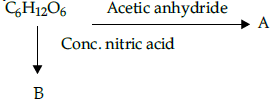(a) A= COOH-(CH2)4-COOH, B= OHC-(CHOCOCH3)4-CH2OCOCH3
(b) A= COOH-(CH2)4 -CHO, B=OHC-(CHOCOCH3)4 -CH2OCOCH3
(c) A= OHC-(CHOCOCH3)3-CH2OCOCH3 B=COOH-(CH2)4 -CHO,
(d) A= OHC-(CHOCOCH3)4-CH2OCOCH3 B=COOH-(CHOH)4-COOH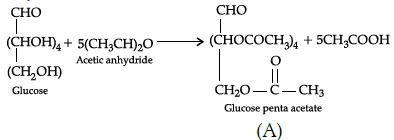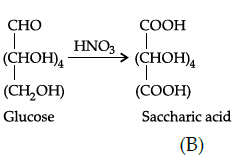Q.37: In lake test for Al3+ ions, there is the formation of coloured ‘floating lake’. It is due to:
(a) Absorption of litmus by [Al(OH)4]-
(b) Absorption of litmus by Al(OH)3
(c) Adsorption of litmus by [Al(OH)4]-
(d) Adsorption of litmus by Al(OH)3

In lake test for Al3+ ions, there is the formation of coloured ‘floating lake’ due to adsorption.

Q.38: A unit cell of NaCl has 4 formula units. Its edge length is 0.50 nm. Calculate the density if molar mass of NaCl = 58.5 g/mol.
(a) 1 g/cm3
(b) 2 g/cm3
(c) 3 g/cm3
(d) 4g/cm3

3 g/cm3
Using formula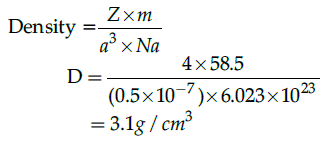Q.39: Which one of the following are correctly arranged on the basis of the property indicated?
(a) I2< Br2<F2<Cl2 [ increasing bond dissociation enthalpy]
(b) H2O > H2S<H2Te<H2Se [increasing acidic strength]
(c) NH3 < N2O< NH2OH<N2O5 [ increasing oxidation state]
(d) BiH3<SbH3<AsH3<PH3<NH3 [ increasing bond angle]

BiH< SbH< AsH< PH< NH3 [ increasing bond angle ] correct order.
(a) I2 < Br2<F2<Cl2 [increasing bond dissociation enthalpy]: incorrect order , correct order is Cl2 > Br2 > F2 > I2.
(b) H2O > H2S<H2Te<H2Se [increasing acidic strength]: incorrect order, correct order is H2O<H2S<H2Se<H2Te
(c) NH3 < N2O< NH2OH < N2O5 [ increasing oxidation state ] : incorrect order NH3 (Oxidation state -3) N2O (Oxidation state +1) NH2OH(Oxidation state -1) N2O5 (Oxidation state +5).

Q.40: What would be the reactant and reagent used to obtain 2, 4-dimethyl pentan-3-ol ?
(a) Propanal and propyl magnesium bromide
(b) 3-methylbutanal and 2-methyl magnesium iodide
(c) 2-dimethylpropanone and methyl magnesium iodide
(d) 2- methylpropanal and isopropyl magnesium iodide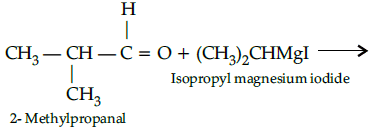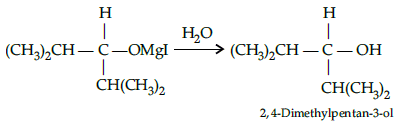Q.41: o-hydroxy benzyl alcohol when reacted with PCl3 gives the product as (IUPAC name)
(a) o- hydroxy benzyl chloride
(b) 2- chloromethylphenol
(c) o-chloromethylchlorobenzene
(d) 4-hydroxymethylphenol

Due to occurrence of the under given reaction: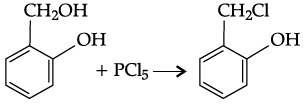Q.42: Which of the following statements is true?
(a) Ammonia is the weakest reducing agent and the strongest base among Group 15 hydrides.
(b) Ammonia is the strongest reducing agent as well as the strongest base among Group 15 hydrides.
(c) Ammonia is the weakest reducing agent as well as the weakest base among Group 15 hydrides.
(d) Ammonia is the strongest reducing agent and the weakest base among Group 15 hydrides.

Ammonia is the weakest reducing agent and the strongest base among Group 15 hydrides.
The reducing character of hydrides increases down the group due to decrease in bond dissociation enthalpy.

Q.43: Identify the secondary alcohols from the following set:

(i) CH3CH2CH(OH) CH3
(ii) (C2H5)3COH
(iii)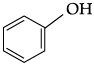(iv)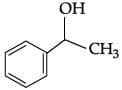(a) (i) and (iv)
(b) (i) and (iii)
(c) (i) and (ii)
(d) (i), (iii)and (iv)

(i) CH3CH2CH(OH)CH3 (secondary)

(ii) (C2H5)3COH (tertiary)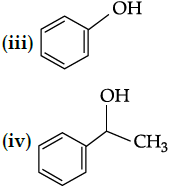Q.44: Alkenes decolourise bromine water in presence of CCl4 due to formation of: (a) Allyl bromide
(b) Vinyl bromide
(c) Bromoform
(d) Vicinal dibromide

CH= CH2 + Br2 → BrCH2 - CH2Br.

Q.45: Directions: In the following questions, A statement of Assertion (A) is followed by a statement of Reason (R). Mark the correct choice as.

Assertion (A): Electron gain enthalpy of oxygen is less than that of Flourine but greater than Nitrogen.
Reason (R): Ionisation enthalpies of the elements follow the order Nitrogen > Oxygen > Fluorine.

(a) Both A and R are true and R is the correct explanation of A.
(b) Both A and R are true but R is NOT the correct explanation of A.
(c) A is true but R is false.
(d) A is false and R is true.

Ionisation enthalpies of the elements follow the order Fluorine >Nitrogen > Oxygen

Q.46: Directions: In the following questions, A statement of Assertion (A) is followed by a statement of Reason (R). Mark the correct choice as.

Assertion (A): Alkyl halides are insoluble in water.
Reason (R) : Alkyl halides have halogen attached to sp3 hybrid carbon.

(a) Both A and R are true and R is the correct explanation of A.
(b) Both A and R are true but R is NOT the correct explanation of A.
(c) A is true but R is false.
(d) A is false and R is true.

Alkyl halides are insoluble in water because they are unable to form hydrogen bonds with water or break preexisting hydrogen bonds.

Q.47: Directions: In the following questions, A statement of Assertion (A) is followed by a statement of Reason (R). Mark the correct choice as.

Assertion(A): Molarity of a solution changes with temperature.
Reason (R): Molarity is a colligative property.
(a) Both A and R are true and R is the correct explanation of A.
(b) Both A and R are true but R is NOT the correct explanation of A.
(c) A is true but R is false.
(d) A is false and R is true.

Molarity is a means to express concentration. It is not a physical property.

Q.48: Directions: In the following questions, A statement of Assertion (A) is followed by a statement of Reason (R). Mark the correct choice as.

Assertion(A): SO2 is reducing agent while TeO2 is an oxidising agent.
Reason(R): Reducing property of dioxide decreases from SO2 to TeO2.
(a) Both A and R are true and R is the correct explanation of A.
(b) Both A and R are true but R is NOT the correct explanation of A.
(c) A is true but R is false.
(d) A is false and R is true.

Q.49: Directions: In the following questions, A statement of Assertion (A) is followed by a statement of Reason (R). Mark the correct choice as.

Assertion (A): Cryoscopic constant depends on nature of solvent.
Reason(R): Cryoscopic constant is a universal constant.
(a) Both A and R are true and R is the correct explanation of A.
(b) Both A and R are true but R is NOT the correct explanation of A.
(c) A is true but R is false.
(d) A is false and R is true.

Cryoscopic constant various with type of solvent.

Section - C

Q.50: Match the following: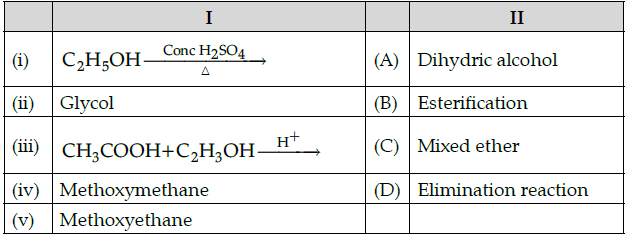Which of the following is the best matched options ?
(a) i-B, v-C, iii-A, iv-D
(b) i-B, ii-A, iii-D, iv-C

(c) i-D, v- C, iii-B, iv-A
(d) i-A, ii-C, iii-D, iv-B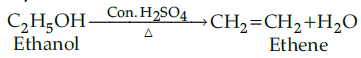Ethanol undergoes elimination reaction when heated with conc. H2SO4 and gives ethene. Glycol - It is dihydric alcohol.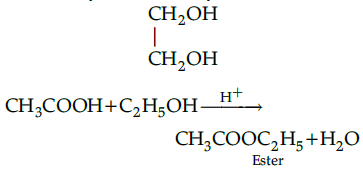Acetic acid reacts with ethanol in acidic medium to form an ester. This reaction is called esterification.
Methoxyethane = CH3OCH2CH3
Since it contains two different alkyl groups so it is called mixed ether.

Q.51: Complete the following analogy:
Glucose: A:: Fructose: B
(a) A: -NH2; B: -CHO
(b) A: >C=O ; B: -CHO
(c) A: -CHO; B: >C=O
(d) A: -CONH2;B: -OH

The functional groups present in glucose and fructose are -CHO and >C=O respectively.

Q.52: Which of the following analogies is correct:

(a) Group 15: Carfon family:: Group 16: Oxygen family
(b) Rhomfic sulphur: α-sulpher:: Monoclinic sulpher: β-sulpher
(c) Caro’s acid: H2SO3:: Oleum: H2S2O7
(d) Halide ion: Cl-:: Pseudohalide ion: OH-

There are two allotropics of sulphur viz Rhombic sulphur (α-sulphur) and Monoclinic sulphur (β-sulphur)

Aryl halides are extremely less reactive towards nucleophilic substitution reactions due to the following reasons:
(i) In haloarenes, the electron pairs on halogen atom are in conjugation with π-electrons of the ring.
(ii) In haloalkane, the carbon atom attached to halogen is sp3 hybridised while in case of haloarene, the carbon atom attached to halogen is sp2 -hybridised.
(iii) In case of haloarenes, the phenyl cation formed as a result of self-ionisation will not be stabilised by resonance.

Q.53: A primary alkyl halide would prefer to undergo ________.
(a) SN1 reaction
(b) SN2 reaction
(c) α-Elimination
(d) Racemisation

A primary alkyl halide would prefer to undergo SN2 reaction

Q.54: What is ‘A’ in the following reaction ?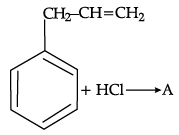(a)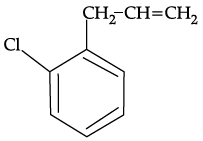(b)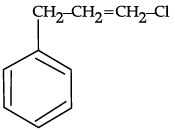(c)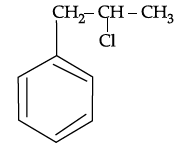(d)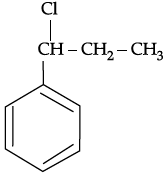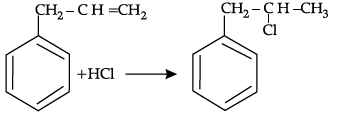Q.55: Which of the following alkyl halides will undergoes SN1 reaction most readily ?
(a) (CH3)3C—F
(b) (CH3)3C—Cl
(c) (CH3)3C—Br
(d) (CH3)3C—I

(CH3)3C-I being a tertiary alkyl halide will most readily undergo SN1 reaction.

The document Class 12 Chemistry: CBSE Sample Question Paper- Term I (2021-22)- 4 Notes | Study Sample Papers for Class 12 Medical and Non-Medical - Class 12 is a part of the Class 12 Course Sample Papers for Class 12 Medical and Non-Medical.
All you need of Class 12 at this link: Class 12Use Code STAYHOME200 and get INR 200 additional OFF

## Sample Papers for Class 12 Medical and Non-Medical

103 docs|4 tests

Track your progress, build streaks, highlight & save important lessons and more!

,

,

,

,

,

,

,

,

,

,

,

,

,

,

,

,

,

,

,

,

,

;